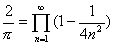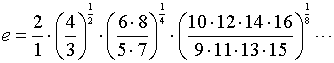### 四、无穷乘积

[基本概念] 设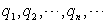=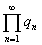, qn0 (n=1,)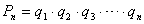Pn=P

P称为无穷乘积的值，记作

P==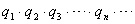, qn0(n=1,)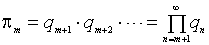[无穷乘积收敛判别法]

(1) 无穷乘积收敛的一个必要条件是：πm=1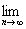qn=1

(2) 无穷乘积收敛的充分必要条件是：级数收敛.L是前面级数的和，则P=eL.

(3) qn=1+an(n=1,2,…)，对充分大的n，若有an>0(an<0)，则=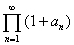收敛的充分必要条件是：级数收敛.

(4) 若级数与级数同时收敛，则=收敛.

(5) 无穷乘积具有零值的充分必要条件是：级数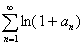的和为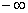.

(6) 无穷乘积绝对收敛的充分必要条件是：级数绝对收敛.

[函数项无穷乘积的一致收敛] 如果函数序列

Pm(x)=(m=1,)[无穷乘积展开式]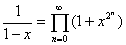(| x| <1)(其中γ为欧拉常数)（其中p跑遍一切素数，ξ(x)称为黎曼ξ函数.）